Courses

# NEET Previous Year Questions: Gravitation- 2

## 11 Questions MCQ Test Physics For JEE | NEET Previous Year Questions: Gravitation- 2

Description
This mock test of NEET Previous Year Questions: Gravitation- 2 for JEE helps you for every JEE entrance exam. This contains 11 Multiple Choice Questions for JEE NEET Previous Year Questions: Gravitation- 2 (mcq) to study with solutions a complete question bank. The solved questions answers in this NEET Previous Year Questions: Gravitation- 2 quiz give you a good mix of easy questions and tough questions. JEE students definitely take this NEET Previous Year Questions: Gravitation- 2 exercise for a better result in the exam. You can find other NEET Previous Year Questions: Gravitation- 2 extra questions, long questions & short questions for JEE on EduRev as well by searching above.
QUESTION: 1

### In a region of only gravitational field of mass `M' a particle is shifted from A to B via three different paths in the figure. The work done in different paths are W1, W2, W3 respectively then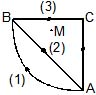[JEE' (Scr.) 2003]

Solution:

Since the gravitational field is a conservative field, hence the work done in taking a particle from one point to another in a gravitational field of path independent.
⟹ w1​=w2​=w3

QUESTION: 2

### What is the minimum energy required to launch a satellite of mass m from the surface of a planet of mass M and radius R in a circular orbit at an altitude of 2R?

Solution:

From conservation of energy,
Total energy at the planet = Total energy at altitude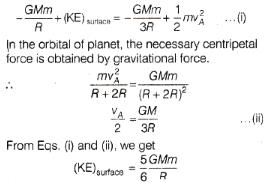QUESTION: 3

### A system of binary stars of masses mA and mB are moving in circular orbits of radii rA and rB respectively. If TA and TB are the time periods of masses mAand mB respectively, then [JEE 2006]

Solution:
QUESTION: 4

A spherically symmetric gravitational system of particles has a mass density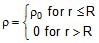where r0 is a constant. A test mass can undergo circular motion under the influence of the gravitational field of particles. Its speed V as a function of distance r (0 < r < ∞) from the centre of the system is represented by

[JEE 2008]

Solution: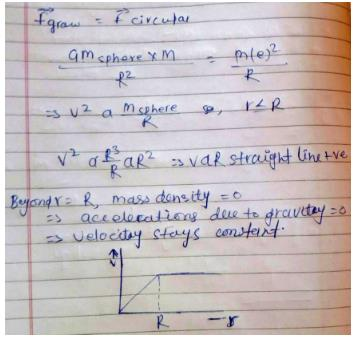QUESTION: 5

STATEMENT-1

An astronaut in an orbiting space station above the Earth experiences weightlessness.

and

STATEMENT-2

An object moving around the Earth under the influence of Earth's gravitaitonal force is in a state of `free-fall'.

[JEE 2008]

Solution:

The space station has an acceleration towards the Earth but it also has a velocity perpendicular to the radius which stops it from falling.

QUESTION: 6

A thin uniform annular disc (see figure) of mass M has outer radius 4 R and inner radius 3R. The work required to take a unit mass from point P on its axis to infinity is

[jee 2010]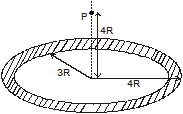Solution: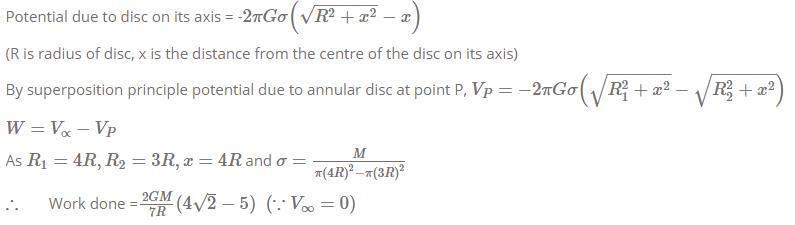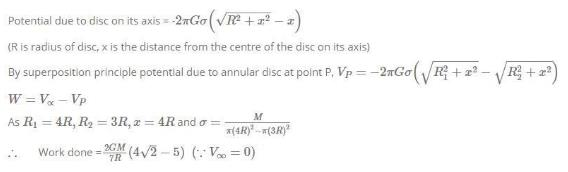*Answer can only contain numeric values
QUESTION: 7

A binary star consists of two stars A (mass 2.2 Ms) and B (mass 11 Ms), where Ms is the mass of the sun. They are separated by distance d and are rotating about their center of mass, which is stationary. The ratio of the total angular momentum of the binary star to the angular momentum of star B about the center of mass is.

[jee 2010]

Solution: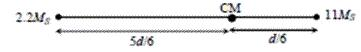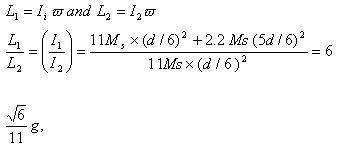*Answer can only contain numeric values
QUESTION: 8

Gravitational acceleration on the surface of a planet is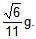where g is the gravitational acceleration on the surface of the earth. The average mass density of the planet is 2/3 times that of the earth. If the escape speed on the surface of the earth is taken to be 11 kms-1, the escape speed on the surface of the planet in kms-1 will be

[jee 2010]

Solution: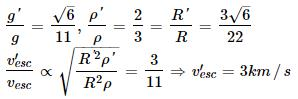QUESTION: 9

A satellite is moving with a constant speed 'V' in a circular orbit about the earth. An object of mass 'm' is ejected from the satellite such that it just escapes from the gravitational pull of the earth. At the time of its ejection, the kinetic energy of the object is

[jee 2011]

Solution:

Orbital speed = √Gm/r
Escape speed = √2Gm/r
So, KE is 2 times

*Multiple options can be correct
QUESTION: 10

Two spherical planets P and Q have the same uniform density , masses Mpand MQ, and surface areas A and 4A, respectively. A spherical planet R also has uniform density and its mass is (MP + MQ). The escape velocities from the planets P, Q and R, are Vp, VQ and VR,respectively. Then

[jee 2012]

Solution:

let mass of planet P = M and radius of planet P = R
Ap​=A
Aq​=4A
∴Rp​=R
And
Rq​=R/2
Also,
density=mass​/volume
mass of planet Q= 8M and radius of planet Q= 2R
mass of planet R= 9M and radius of planet R =91/3​R
so, escape velocity of P =Vp​=√2GM​​/R
VQ​=√2G8M/2R ​​=2VP
VR​= √2G8M​​/91/3​R =91​/3 VP​
so VR​>VQ​>VP
and VQ/ VP​​​=2

*Multiple options can be correct
QUESTION: 11

Two bodies, each of mass M, are kept fixed with a separation 2L. A particle of mass m is projected from the midpoint of the line joining their centres, perpendicular to the line. The gravitational constant is G. The correct statement(s) is (are)

[jee 2013]

Solution: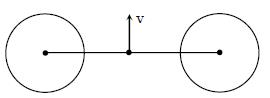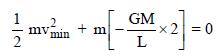Energy conservation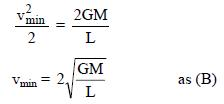total energy of mass does not remain constant as net force on it is non-zero